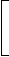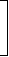# Topic: Basic Numeracy (Test 5)

Topic: Basic Numeracy
Q.1
If one-third of one-fourth of a number is 15, then three-tenth of that number is:
A. 35
B. 36
C. 45
D. 54
Explaination / Solution:

Let the number be x.

 Then, 1 of 1 of x = 15x = 15 x 12 = 180. 3 4

 So, required number =3 x 180= 54. 10

Workspace
Report
Q.2
What decimal of an hour is a second ?
A. .0025
B. .0256
C. .00027
D. .000126
Explaination / Solution:

 Required decimal = 1 = 1 = .00027 60 x 60 3600

Workspace
Report
Q.3
The cube root of .000216 is:
A. .6
B. .06
C. 77
D. 87
Explaination / Solution:

 (.000216)1/3 =2161/3 106

 =6 x 6 x 61/3 102 x 102 x 102

 = 6 102

 = 6 100

= 0.06

Workspace
Report
Q.4
Two numbers are respectively 20% and 50% more than a third number. The ratio of the two numbers is:
A. 2 : 5
B. 3 : 5
C. 4 : 5
D. 6 : 7
Explaination / Solution:

Let the third number be x.

 Then, first number = 120% of x = 120x = 6x 100 5

 Second number = 150% of x = 150x = 3x 100 2Ratio of first two numbers =6x : 3x= 12x : 15x = 4 : 5. 5 2

Workspace
Report
Q.5
Three pipes A, B and C can fill a tank from empty to full in 30 minutes, 20 minutes, and 10 minutes respectively. When the tank is empty, all the three pipes are opened. A, B and C discharge chemical solutions P,Q and R respectively. What is the proportion of the solution R in the liquid in the tank after 3 minutes?
A. 5/11
B. 6/11
C. 7/11
D. 8/11
Explaination / Solution:

 Part filled by (A + B + C) in 3 minutes = 31 + 1 + 1=3 x 11= 11 . 30 20 10 60 20

 Part filled by C in 3 minutes = 3 . 10Required ratio =3 x 20= 6 . 10 11 11

Workspace
Report
Q.6
A vessel is filled with liquid, 3 parts of which are water and 5 parts syrup. How much of the mixture must be drawn off and replaced with water so that the mixture may be half water and half syrup?
A. 1/3
B. 1/4
C. 1/5
D. 1/7
Explaination / Solution:

Suppose the vessel initially contains 8 litres of liquid.

Let x litres of this liquid be replaced with water.

 Quantity of water in new mixture =3 - 3x + xlitres 8

 Quantity of syrup in new mixture =5 - 5xlitres 83 - 3x + x=5 - 5x8 85x + 24 = 40 - 5x10x = 16x = 8 . 5

 So, part of the mixture replaced =8 x 1= 1 . 5 8 5

Workspace
Report
Q.7
In a 100 m race, A can give B 10 m and C 28 m. In the same race B can give C:
A. 18 m
B. 20 m
C. 27 m
D. 9 m
Explaination / Solution:

A : B = 100 : 90.

A : C = 100 : 72.

 B : C = B x A = 90 x 100 = 90 . A C 100 72 72

When B runs 90 m, C runs 72 m.

 When B runs 100 m, C runs72 x 100m = 80 m. 90B can give C 20 m.

Workspace
Report
Q.8
Tickets numbered 1 to 20 are mixed up and then a ticket is drawn at random. What is the probability that the ticket drawn has a number which is a multiple of 3 or 5?
A. 1/2
B. 2/5
C. 8/15
D. 9/20
Explaination / Solution:

Here, S = {1, 2, 3, 4, ...., 19, 20}.

Let E = event of getting a multiple of 3 or 5 = {3, 6 , 9, 12, 15, 18, 5, 10, 20}.P(E) = n(E) = 9 . n(S) 20

Workspace
Report
Q.9
The banker's discount on a bill due 4 months hence at 15% is Rs. 420. The true discount is:
A. Rs. 400
B. Rs. 360
C. Rs. 480
D. Rs. 320
Explaination / Solution:

T.D.
 = B.D. x 100 100 + (R x T)
= Rs.420 x 100100 +15 x 13
 = Rs.420 x 100105
= Rs. 400.

Workspace
Report
Q.10
A train running at the speed of 60 km/hr crosses a pole in 9 seconds. What is the length of the train?
A. 120 metres
B. 180 metres
C. 324 metres
D. 150 metres
 Speed=60 x 5m/sec =50m/sec. 18 3Length of the train =50 x 9m = 150 m. 3